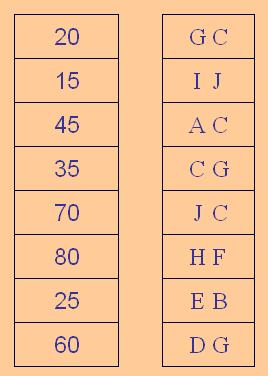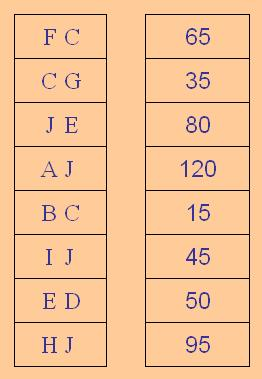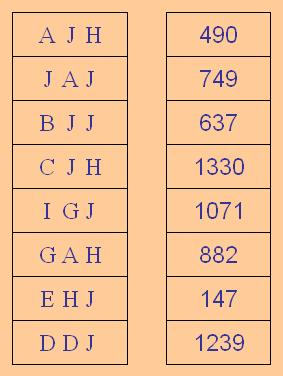#### You may also like### Percentage Unchanged

If the base of a rectangle is increased by 10% and the area is unchanged, by what percentage is the width decreased by ?### Circle Packing

Equal circles can be arranged so that each circle touches four or six others. What percentage of the plane is covered by circles in each packing pattern? ...### Round and Round

Prove that the shaded area of the semicircle is equal to the area of the inner circle.

# A Change in Code

##### Age 14 to 16Challenge Level

All numbers in the first column have been increased by the same percentage, to give the results shown in the second column.

But the results have been disguised - their digits are replaced by letters.

A given letter stands for the same numeral every time it appears in that column, so for example, whatever the C stands for, it stands for that numeral every time the C appears.

Can you work out the percentage increase?Notice that all the numbers in the first column are multiples of 5, and all the numbers in the second column are whole numbers.

Notice that when 80 is increased by the secret percentage, the result is a two-digit number.

It's a new coding - the letters now stand for different numerals.

What percentage have the numbers in the first column been increased by this time?Notice that all the numbers in the second column are multiples of 5.

Notice that when the numbers in the first column are increased by the secret percentage, the result is almost always a two-digit number.

Can you work out the percentage increase here?

Different coding again, and much harder...Do the numbers in the second column have a common factor?